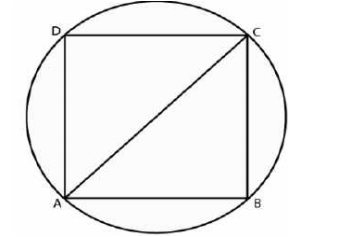# ML Aggarwal Solutions Class 9 Mathematics Solutions for Mensuration Exercise 16.3 in Chapter 16 - Mensuration

A circular field has a perimeter of 660 m. A plot in the shape of a square having its vertices on the

the circumference is marked in the field. Calculate the area of the square field.

It is given that

The perimeter of circular field = 660 m

The radius of the field = 660/2 π

Substituting the values

= (660 × 7)/ (2 × 22)

= 105 m

Here

ABCD is a square which is inscribed in the circle where AC is the diagonal which is the diameter of the circular

fieldConsider a as the side of the square

AC = √2 a

a = AC/√2

Substituting the values

a = (105 × 2)/ √2

Multiply and divide by √2

a = (105 × 2 × √2)/ (√2 × √2)

By further calculation

a = (105 × 2 × √2)/ 2

a = 105 √2 m

We know that

\text { Area of the square }=\mathrm{a}^{2}

\begin{aligned} &\text { It can be written as }\\ &=(105 \sqrt{2})^{2}\\ &=105 \sqrt{2} \times 105 \sqrt{2}\\ &=22050 \mathrm{m}^{2} \end{aligned}

Video transcript
"hello and welcome my dear students i am surprised the maths would run leader and i am here with a new question it is a circular field as a perimeter of 660 meter a plot in the shape of a square having its vertices on this circumference is marked in the field calculate the area of the square field so without wasting much time let's have a look at the solution and before that we look at the important points of this question so the we know that a circular field has a perimeter of 660 meters so the perimeter is 660 meters also we observe that our plot is in the shape of a square having its vertices on the circumference so let's look at the solution so we have been given that perimeter i'm writing p for perimeter it's nothing but a 660 meter and we know that the perimeter of a circle is nothing but 2 pi r which is equal to 660 meter which further implies that r is nothing but equal to 660 by 2 pi so further solving it we get 660 into 7 upon 2 into 22 you put the value of the pi which is 22 by 7 we get 105 meter also we observe that if it is a square which is inscribed in the circle where ac is the diagonal which is the diameter of the circular field circular field so i've written sirc that means circular field let's consider the side of square ba so let's decide of square ba so we know that ac is nothing but equals to root 2a using the pythagoras theorem we know that both the sides of the square are equal we get a diagonal as root 2 into a which further implies that the side that is a is equals to ac by root 2. so we'll substitute the values so we get a as 105 into 2 by root 2 because 105 is the radius and ac is the diameter of the circle so by further calculation we get 105 into 2 into root 2 upon 2 which further implies that a is equals to 105 root 2. now the area of square is equals to side square this we all know and side is nothing but a square so we'll simply substitute the value so we get 105 root 2 square which is nothing but equals to 22 50 meter square that is double two zero five zero meter square so i hope my students got this question well do not forget to hit the subscribe button you can also share your doubts in the comment section see you next time bye"
Related Questions

Lido

Courses

Teachers

Book a Demo with us

Syllabus

Maths
CBSE
Maths
ICSE
Science
CBSE

Science
ICSE
English
CBSE
English
ICSE
Coding

Terms & Policies

Selina Question Bank

Maths
Physics
Biology

Allied Question Bank

Chemistry
Connect with us on social media!